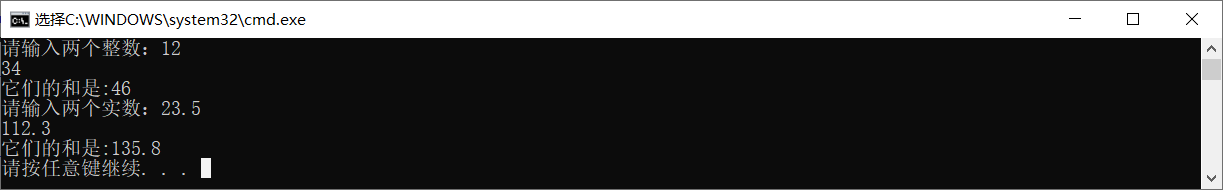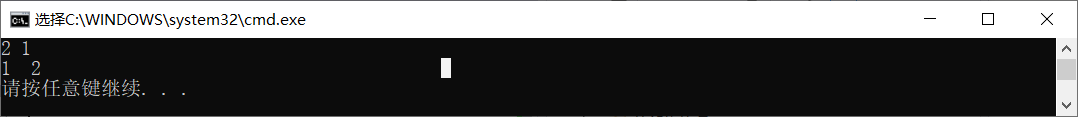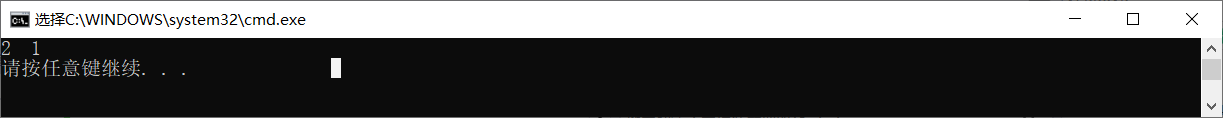# C++面向对象——第二次作业

## 一、为什么要用函数？

1、使程序中的代码模块化、相对独立，方便快速重复调用一串代码。
2、减少代码量，界面看起来简洁，增强代码的可读性
3、便于调试、修改和维护

#include <iostream>

using namespace std;

void swap(int *a, int *b)
{
int temp;
temp = *a;
*a = *b;
*b = temp;
}

int main()
{
int a;
int b;
cout << "请输入a,b的值：" << endl;
cin >> a >> b;
swap(a, b);
cout << "a=" << a << "   b=" << b<<endl;
return 0;
}


## 二、为什么要用函数重载？

#include <iostream>

using namespace std;

{
return x + y;
}

{
return x + y;
}

int main()
{
int a;
int b;
cout << "请输入两个整数：";
cin >> a >> b;
cout << "它们的和是:" << add(a, b) << endl;

double c;
double d;
cout << "请输入两个实数：";
cin >> c >> d;
cout << "它们的和是:" << add(c, d) << endl;
}### 总结

1、函数的参数个数不同。
2、函数的参数类型不同或者参数类型顺序不同。

## 三、什么是值传递？

 void swap1(int p, int q)
{
int temp;
temp = p;
p = q;
q = temp;
cout << p << " " << q << endl;
}
int main()
{
int a = 1, b = 2;
swap1(a, b);
//swap2(&a,&b);
//swap3(a,b);
cout << a << "  " << b << endl;
return 0;
}## 四、什么是地址传递？

void swap2(int *p, int *q)
{
int temp;
temp = *p;
* p = *q;
* q = temp;
}
int main()
{
int a = 1, b = 2;
//swap1(a, b);
swap2(&a,&b);
//swap3(a,b);
cout << a << "  " << b << endl;
return 0;
}### 总结

1、调用者让被调者修改自己空间的值
2、用在连续空间的传递
（三、四参考于<值传递与地址传递（C语言）>）

## 五、设计实验教案，分析如何编写递归函数？

1、递归表达式
2、结束条件

n！的递归

#include <iostream>

using namespace std;
int J(int n);
int J(int n)
{
if (n == 1)
return 1;
else
return n*J(n - 1);
}
int main()
{
int a;
cout << "请输入a：" ;
cin >> a;
J(a);
cout << "a的阶乘是：" << J(a);
}


### 总结

1、为防止递归无休止的调用，递归必须设置递归终止条件
2、汉诺塔、菲波那切数列用递归更简单
3、递归虽然简化程序，但降低程序执行效率，一个字，慢

posted @ 2019-09-16 22:37  小囡囡不成仙  阅读(126)  评论(0编辑  收藏  举报